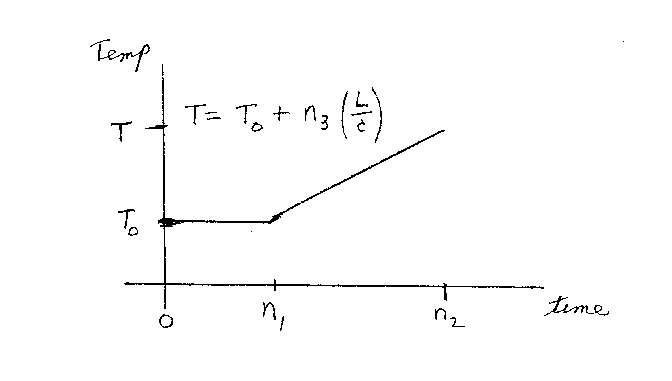Problem D20: A substance starts out as a solid at temperature T0. Heat is added at a constant rate. The temperature of the substance is shown in the graph below. For the first n1 seconds the substance melts. From time n1 to time n2 the substance is in the liquid state. The temperature at time n2 is T = T0 + n3 L/c, where L is the latent heat of fusion and c is the specific heat capacity for the liquid state. L/c has the same units of temperature as T0 and T. what is n3? Note that n1 and n2 have the same units of time, and n3 is unitless.n1 = n2 = Input n3:

If you are currently in my class, you can record your grade by entering your name and student ID number (without the leading zeros) below and clicking on "record grade".
 First Name = Last Name = ID = Problem: